Donate

``````

Introduction to Angles: The Art of Problem Solving:

Angles

Angles are used in daily life.  Engineers and architects use angles for designs, roads, buildings and sporting facilities.  Athletes use angles to enhance their performance.  Carpenters use angles to make chairs, tables and sofas.  Artists use their knowledge of angles to sketch portraits and paintings.
If two lines meet (or intersect) at a point, then an angle is formed.  The point of intersection of the lines is called the vertex.

An angle is shown below.Lines AB and AC meet at the point A to form an angle.  The point A is the vertex of the angle, and the lines that meet to make the angle are called the arms of the angle.

Naming Angles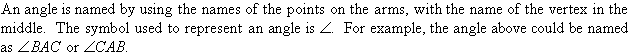Size of an Angle

The amount of turn from one arm of the angle to the other is said to be the size of an angle.The size of an angle is measured in degrees; and the symbol used to represent degree is º.  There are 360º in a full turn (or circle).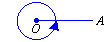Note:

degree is defined such that the angle of one full turn (or circle) is 360 degrees.

Measuring Angles

protractor is used to measure angles.  In this section, we will consider the use of a protractor that has the shape of a semi-circle and two scales marked from 0º to 180º.The two scales make it easy for us to measure angles facing different ways.To measure the size of angle ABC, place the protractor over the angle so that the centre of the protractor is directly over the angle’s vertex, B; and the base line of the protractor is along the arm, BA, of the angle.We use the inner scale to measure the angle ABC, as the arm AB passes through the zero of the inner scale.  Following the inner scale around the protractor, we find that the other arm, BC, passes through the inner scale at 60º.  So, the size of angle ABC is 60 degrees. We write this as follows: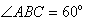To measure the size of angle PQR, place the protractor over the angle so that the centre of the protractor is directly over the angle’s vertex, Q; and the base line of the protractor is along the arm, PQ, of the angle.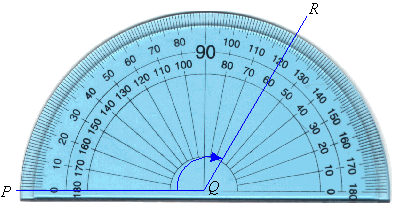We use the outer scale to measure the angle PQR, as the arm PQ passes through the zero of the outer scale.  Following the outer scale around the protractor, we find that the other arm, QR, passes through the outer scale at 120º.  So, the size of angle PQR is 120 degrees.  We write this as follows: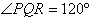Types of Angles

Angles are classified according to their size.
An acute angle is greater than 0º and less than 90º.right angle equals exactly 90º.Note that a right angle is marked on the diagram as a small square.
An obtuse angle is greater than 90º and less than 180º.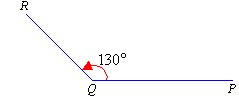A straight angle equals exactly 180º.reflex angle is greater than 180º and less than 360º.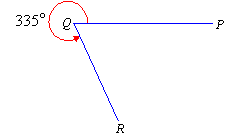perigon (or a revolution) is an angle that equals exactly 360º.Measuring Reflex Angles

Recall that:

A protractor can be used to measure the size of an acute angle (between 0º and 90º) and an obtuse angle (between 90º and 180º).
Now, we will use a protractor to measure the reflex angle PQR.To measure the reflex angle PQR, extend the arm PQ to A to form angle PQA which is a straight angle.  Then measure the size of the angle AQR and add 180º.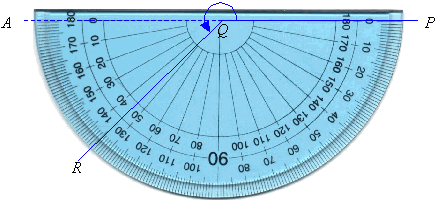The Story of Maths : The Genius of the East /قصة الرياضيات : عبقرية الشرق

This is the untold story of the mathematics of the East, that transformed the West.

Why Math?

To put it plainly math exists in our imagination. When we practice difficult mathematics we improve our ability to utilize imagination. We live in a universe bathed in order, or at least it appears this way to us. This order takes on the form of mathematical logic in our brain. If you learn the art of math I promise your interpretation of reality will be altered and improved forever.

Placing Numbers On A Number Line

Use the number line for adding and subtracting integers:

• Add a positive integer by moving to the right on the number line
• Add a negative integer by moving to the left on the number line
• Subtract an integer by adding its opposite

Watch out! The negative of a negative is the opposite positive number. That is, for real numbers, – (- a ) = + a

Here are the steps for adding fractions with different denominators. We will break-down each step just like before to make sure you’ve got it.

So, here are the steps.
1. Build each fraction so that both denominators are equal.Remember, when adding fractions with different denominators, the denominators must be equal. So we must complete this step first.
2. Re-write each equivalent fraction using this new denominator
3. Now you can add the numerators, and keep the denominator of the equivalent fractions.
4. Re-write your answer as a simplified or reduced fraction, if needed.

We know this sounds like a lot of work, and it is, but once you understand thoroughly how to find the Common Denominator or the LCD, and build equivalent fractions, everything else will start to fall into place. So, let’s take our time to do it Right!

But keep in mind, if you are doing homework, be sure to answer the problems in the form asked for in the assignment.

+

Notice that the overall size of our point of reference
(The Whole) is EXACTLY the same.

Step #1 in our rule tells us that the denominators must be equal. And the easiest way to find a common denominator is to just multiply the denominators.

So let’s do that now…

2 x 3 = 6

The Common Denominator for 1/2 and 1/3 is 6

Step #2 – Re-write each equivalent fraction using this new denominator.

Since…

And…

1/3 is equivalent to 2/6

We re-write our equation to read…

Now we are ready to do Step #3 – ADD the numerators, and keep the denominator of the equivalent fractions (which is 6).

So, we end up with…

=

Finally, Step #4 – Re-write your answer as a simplified or reduced fraction, if needed.

In our example, the answer (5/6) is already in its simplest form. So, no further action is required!

That’s It!

quick and easy way to add fractions with different denominators.

The Fundamental Theorem of Arithmetic

The Basic Idea is that any integer above 1 is either a Prime Number, or can be made by multiplying Prime Numbers together.